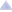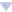Please subscribe to the official Codeforces channel in Telegram via the link https://t.me/codeforces_official. ×

### dutin's blog

By dutin, history, 2 years ago,I've seen CF tutorials for many other sections of CSES but didn't see one for strings, so I thought of writing one. Constructive criticism (or just regular criticism) is always welcome!

Note that there are many ways to do a problem. For instance, linear time string searching can be done with hashing, KMP, Z-Algorithm, Aho-Corasick Automaton, etc. . I used the way that was most intuitive for me, but there might exist other ways that have shorter code.

1. Word Combinations

Solution
Code

2. String Matching

Solution
Code

3. Finding Borders

Solution
Code

4. Finding Periods

Solution
Code

5. Minimal Rotation

Solution
Code

6. Longest Palindrome

Solution
Code

7. Required Substring

Solution
Code

Solution and Code from toniskrijelj

8. Palindrome Queries

Solution
Code

9. Finding Patterns

Solution
Code

10. Counting Patterns

Solution
Code

11. Pattern Positions

Solution
Code

12. Distinct Substring

Solution
Code

13. Repeating Substring

Solution
Code

14. String Functions

Solution
Code

15. Substring Order I

Solution
Code

16. Substring Order II

Solution
Code

17. Substring Distribution

Solution
CodeComments (21)
| Write comment?
 » 2 years ago, # | ← Rev. 2 →   I thought I ought to mention that Substring Order 1, Substring Order 2, and Substring Distribution all have a suffix array + lcp solution as well.Substring Order 1Consider the solution for counting all the unique substrings, you take the sum of all lcp[i]. In the same manner you can loop over all the unique substrings in lexicographical order. Consider the suffix array, if we look at all substrings that start at the index of the start of the suffix (or sa[i]), the ones that have already be counted are the substrings in the longest common prefix of the current suffix and the suffix before it. This is by the definition of lcp[i]. So you can do pie and get that the amount of unique substrings sa[i] contributes is n — sa[i] — lcp[i] (with some off by 1's of course). A scuffed implementation can be found here.Substring Order 2This solution revolves around the fact that the sum of lcp[i] is linear. There are two cases to consider: 1) the answer substring appears more than once in the string, 2) the answer substring does not. For case 2, we can refer to the solution for Substring Order 1, but for case 1, its a little harder. So for some sa[i] we have all the substrings that start at sa[i] and have length <= lcp[i]. Let's try to think about how many times exactly each of these strings appear. Since we have lcp(i, h) >= lcp(i, j) for any i < h < j (and lcp returns the length of the longest common prefix of the two suffixes), we can see that binary search can be done on how many times this substring appears. We start at index sa[i] and binary search on the last time lcp(sa[i], sa[j]) >= the length of the substring we are looking at. What is the complexity of such an algorithm? It is actually still O(nlogn). To avoid double counting, we should only count the contribution of a string if and only if lcp[i] >= lcp[i-1] (since that means its the first time the substring is lexicographically smallest). For the rest of the substrings, we can use the n — sa[i] — lcp[i] trick from Substring Order 1. I am not even going to try and say this implementation is good, but here it is.Substring DistributionThis problem again relies on the fact that the contribution of unique strings from some suffix sa[i] is n — sa[i] — lcp[i]. So for some given suffix it will contain unique substrings of lengths lcp[i]+1 to sa[i]. Using the psum[l]++, psum[r]-- trick, we have an algorithm with linear complexity with the suffix array construction aside. A scuffed implementation can be found here.
•  » » My implementation of your solution of Substring Order II : https://cses.fi/paste/1a1adab9babc8ef84a7c56/ I guess it might be more readable and easy to understand. (I wasn't able to understand your approach, until I did a dry run on your code. So I am putting my implementation which might be easier to understand)
•  » » The idea that the sum max(lcp[i] — lcp[i-1], 0) is linear that you present in the substring order II is wrong. Kasai algorithm for building the lcp array is linear, but it acts on suffixes in decreasing order of size while your algorithm acts on suffixes in lexicographical order. I coded the solution that you described and got AC on CSES, but than I hacked both our solutions with a random string concatenated with itself, which achieves the worst time complexity of O(n²log(n)) (n² different substrings that appear at least twice and we do a binary search on the lcp sparse table for each one).
 » Auto comment: topic has been updated by dutin (previous revision, new revision, compare).
 » I need solution problem special string. Can you help me?
•  » »
•  » » » Thanks you so much. But it only have code, you can give me tutorial for this problem
•  » » » » This problem can be seen as an extension of prefix sums. Run a prefix sum on the string, keeping track of an array of length 26 which is the number of occurrences of each letter. If the current prefix contains all letters in the string and each letter occurs an equal number of times, it is a valid substring. Because each substring can be represented as a differences of prefixes, we find the number of previous prefixes where the excess elements are in excess the exact same amount. For instance, at the current prefix, we have: a: 4 b: 3 c: 2 d: 2 e: 2 ...We have to find the number of previous prefixes where a is +2 and b is +1. a: +2 b: +1 c: 0 d: 0 e: 0 ...This is where having a map comes in handy. The mentioned solution uses a map of vectors, although an array would probably work better.
•  » » » :O
 » 2 years ago, # | ← Rev. 2 →   7. Required Substring: Solution:We build string character by character, and we track progress of building required string as our substring.Example:Required substring: ABCCurrent string: XHG ABHere our progress equals 2, because last two characters match with first two characters of required substring.Now we only need a way to recalculate progress as we push more characters into our string, and for that we will use KMP. If current pushed character equals t[progress] we increase progress by one. If it reaches t.size(), we now have t as substring and rest can be filled however, but, if we pushed character that doesn't equal to current progress character, we must decrease progress value. Naively, you'd think it resets to 0, but look at this:Required substring: ABACCurrent string: ABA and we insert B (ABAB, progress before inserting is 3 because ABA is matched)Current string will be ABAB and its progress will be 2, as suffix "AB" matches prefix of 2 characters of required substring.So it doesn't necessary resets to 0, we will use KMP to calculate what it resets to, and we continue forward to build rest of the string.If we filled all n positions without reaching progress of t.size(), we doesn't add to answer. Code:#include #include using namespace std; typedef long long ll; const int mxN = 1001; const int mxM = 101; const int mxK = 26; const int mod = 1e9+7; int kmp[mxN]; void calc(string &s) { kmp = 0; for(int i = 1; i < (int)s.size(); i++) { int trymatch = kmp[i-1]; while(true) { if(s[trymatch] == s[i]) { kmp[i] = trymatch+1; break; } else if(trymatch) { trymatch = kmp[trymatch-1]; } else { kmp[i] = 0; break; } } } } ll dp[mxN][mxM]; bool done[mxN][mxM]; ll solve(int i, int n, int j, string &s) { if(done[i][j]) return dp[i][j]; done[i][j] = true; if(i == n) return dp[i][j] = (j==(int)s.size()?1:0); if(j == (int)s.size()) { return dp[i][j] = (mxK*solve(i+1,n,j,s))%mod; } ll ans = 0; int t; for(int k = 0; k < mxK; k++) { t = j; while(true) { if(k == s[t]-'A') { t++; break; } else if(t) { t = kmp[t-1]; } else break; } ans += solve(i+1,n,t,s); ans %= mod; } return dp[i][j] = ans; } int main() { ios_base::sync_with_stdio(0); cin.tie(0); int n; cin >> n; string s; cin >> s; calc(s); cout << solve(0, n, 0, s); } 
•  » » Thanks for the solution! Do you mind if I attribute this to you and move this up to the main post?
•  » » » You can post it, of course :)
 » All of those are solvable using hashing, except the first one, which has a simpler solution using Trie. ShortA simpler solution to the first problem. #include using namespace std; const int N = (int) 1e6 + 9; const int mod = (int) 1e9 + 7; int T[N], id = 1, dp[N]; bitset endT; int main() { string s; int k; cin >> s >> k; int n = s.length(); for (int i = 0; i < k; i++) { string t; cin >> t; int cr = 0; for (char& c : t) { c -= 'a'; if (!T[cr][c]) T[cr][c] = id++; cr = T[cr][c]; } endT[cr] = 1; } dp[n] = 1; for (int i = n - 1; i >= 0; i--) { s[i] -= 'a'; int cr = 0; for (int j = i; j < n && T[cr][s[j]]; j++) { cr = T[cr][s[j]]; if (endT[cr]) { dp[i] = (dp[i] + dp[j + 1]) % mod; } } } cout << dp << '\n'; return 0; } P.S. Maybe except the 7the one also, I'm not sure.
•  » » How to solve Finding Periods with hashing?
•  » » » Using polynomial hashes we can get the hash of any substring in $\mathcal{O}(1)$ time. We can naively check each prefix, and a prefix of length $k$ takes time $\frac n k$ time to check. Thus, the problem can be solved in $\mathcal{O}(n \ln n)$ time.
•  » » » » I didn't know we could get the hashes in $O(1)$, thank you.
•  » » Is the 17. Substring Distribution solvable by hashing? Naively checking all substrings of lengths from 1 to n — 1 is going to result in $O(n ^ 2)$ time complexity, unless there is some other way?
 » Can you explain why using Z-algorithm can solve Finding Periods? I don't know how to guide to the conclusion that checking i + z[i] >= n is sufficient.
•  » » If the suffix starting at $i$ is equal to the prefix of the string up to $i$, then $s[:i-1]$ is a period. You can verify that this works on the sample case ($s="abcabca"$, $z=[0,0,0,4,0,0,1]$). I should have mentioned that the string itself is always a period and can be hardcoded.
•  » » » Thanks for your reply! I early found that I was stuck in the point that I think the substring between the same string can't be generated by period. But I finally realized that it was part of the period, it was just being cut off.
 » 4 days ago, # | ← Rev. 2 →0.ComplaintFrame { display: inline-block; position: absolute; top: 0; right: -1.4em; } .ComplaintFrame a { text-decoration: none; color: #ff8c00; opacity: 0.5; } .ComplaintFrame a:hover { opacity: 1; } ._ComplaintFrame_popup p, ._ComplaintFrame_popup button { margin-top: 1rem; } ._ComplaintFrame_popup input[type=submit] { padding: 0.25rem 2rem; } ._ComplaintFrame_popup ul { margin-top: 1em !important; margin-bottom: 1em !important; } Can anyone help me with repeating substring? https://cses.fi/paste/eb1576bc1900b84776a439/ I don't know why I got wrong using binary search + hash. It should work?